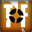Team Fortress 2

### Team Fortress 2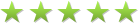930 ratings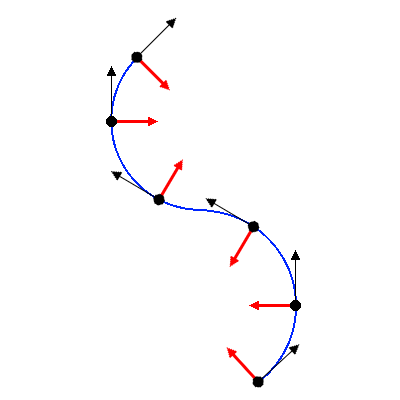More Than You Ever Wanted to Know About Air Strafing
By Kered13
This guide provides a mathematical description of air strafing, explaining why this technique works (and others do not).

This guide first provides a description of the "rules" that the Source engine uses for air movement. From this it derives an explanation for why air strafing works, and briefly discusses some different ways to air strafe. It also attempts to dispel some myths in the process. Later the guide goes into much deeper into math, providing exact formulas for air movement and drawing some more precise conclusions.Award
The Basics
In TF2 movement, there are three vectors that we are primarily concerned with:
-Orientation (A unit vector in the direction we are facing)
-Velocity (A vector with units Hammer Units per second (HU/s) in the direction of movement)
-Acceleration (A vector with units HU/s^2 in the direction of acceleration)

The acceleration vector is determined by our orientation and movement keys. Specifically, the movement keys determine our acceleration relative to our orientation, as shown in the figure. (Note: This guide will assume you use WASD for movement.)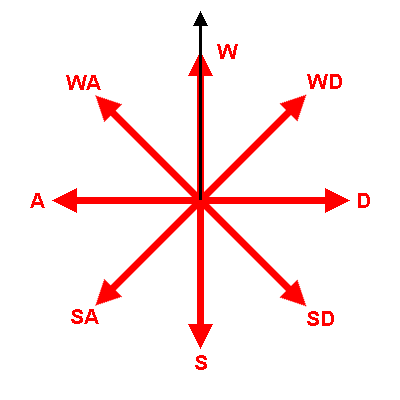The velocity is updated every logical frame by adding the acceleration vector, scaled by the frame time, to the velocity vector: v' = v + t*a. The logical framerate is 66 FPS, giving t=.0152. Note that the logical framerate is unrelated to the graphics framerate.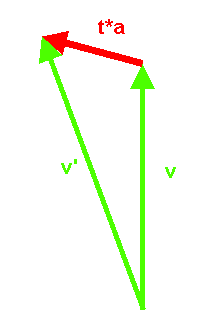Air acceleration in TF2 is equal to 10 times your maximum ground speed, so for example, the air acceleration for the Heavy is 2300 HU/s^2, and for the Scout is 4000 HU/s^2. [Note 1]

Pretty simple so far.
The Speed Limit
So if acceleration is added to velocity, why can't we just hold W and accelerate for ever? That's because in the Source engine, there is an Air Speed Limit. The game prevents air acceleration above this limit. In Team Fortress 2, that limit is...

30 HU/s

We will write this limit as L. To put this in perspective, a spun up brass beast Heavy moves at 44 HU/s. Okay, but if the speed limit is so slow, then how can we move faster than 30 HU/s in the air? Well there is an explanation. You see, the Source engine doesn't slow you down when you're over the speed limit. If that were the case, then you couldn't carry your momentum through the air when you got blasted. It also doesn't prevent all acceleration while you're over the speed limit, if it did that then you would have no control in the air, you wouldn't even be able to stop!

Here is what the Source engine does do: Before applying acceleration each frame, the engine checks the projection of your velocity onto your acceleration. If you're not familiar with vector projection, take a moment to read the Wikipedia article[en.wikipedia.org]. Given an an angle θ between the velocity vector v and the acceleration vector a, we can calculate the magnitude of the projection of v onto a with the equation |v proj a| = |v|cos(θ).

If the projection is greater than the speed limit, then the acceleration is completely ignored. If it is less than the speed limit, then the acceleration is applied, but only up to the speed limit, thus ensuring that v' proj t*a <= L, where v' is the new velocity.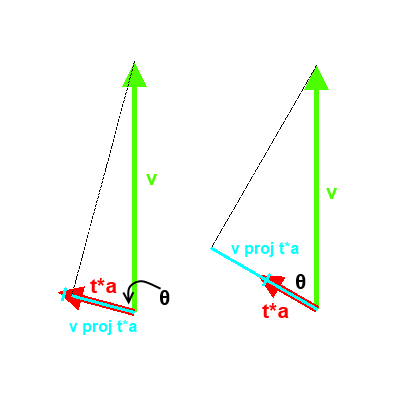The examples above demonstrate this. Here the projection is shown as a teal line, while the limit is a teal cross bar. In the example on the left, the projection is less than L, so the acceleration vector will be applied (but only up to the limit). In the example on the right, the projection is greater than L, so no acceleration is applied.

The complete equation for v' is therefore:

v' = if |v proj a| < L - |t*a|: v + t*a if L - |t*a| <= |v proj a| < L: v + (L - |v|cos(θ))*a/|a| if L <= |v proj a|: v

But remember that the speed limit is very small, 30 HU/s, while movement speeds typically range from 230 to 400 HU/s. This means that any forward, even just slightly forward, acceleration will be cutoff. This is why we can't just hold W and go flying through the air. So if we want our acceleration to do anything, it will need to be perpendicular to or behind our velocity.
Air Strafing
If we want our acceleration to do something, we can calculate the minimum angle between velocity and acceleration such that we stay under the speed limit:

L >= |v|cos(θ) θ >= acos(L/|v|)

For the Scout, with 400 HU/s, this gives 86 degrees. For the Heavy's, with 230 HU/s, we get 83 degrees. But this is only the angle at which we get any acceleration at all. In practice we want to get as much acceleration as possible. The minimum angle in this case is given by:

L - |t*a| >= |v|cos(θ) θ >= acos((L - |t*a|)/|v|)

For the Scout, at 400 HU/s, this gives 94 degrees, and for the Heavy, at 230 HU/s, 91 degrees. This means that we don't actually get the full acceleration until we are accelerating slightly /behind/ our velocity. It turns out this is true for all classes at all speeds, because L - |t*a| < 0 for all classes. But the difference from true perpendicular is small enough that we can ignore it, for now.

So we can't accelerate forwards, but we can accelerate perpendicular to our velocity. When we do this we turn a little bit, which is good, but now we have a problem: The projection of our new velocity onto our acceleration is at the speed limit. This prevents us from turning any more. How can we fix this? We need to turn our acceleration so that it is perpendicular to our velocity again. Since this is a very small angle, we can only do this by turning our mouse slightly to the left. Now our acceleration is perpendicular to our velocity again, and we turn some more.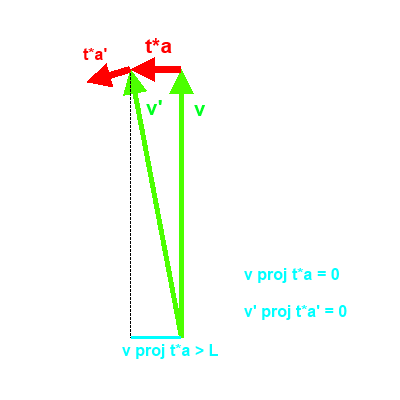This repeated process, which gradually turns us in the air, is what we call air strafing. And now we have derived the most important principle of air strafing: Always keep acceleration perpendicular to velocity.
A and D Strafing
One of the most confusing things for new players in TF2 is learning not to hold W in the air, at least while airstrafing. This seems counter-intuitive, we hold W to go forwards, right? Now we have laid the foundation to understand why holding W is counter-productive.We established above that in order to airstrafe, we need to keep our acceleration perpendicular to our velocity. We also learned at the beginning that the direction of acceleration is a function of our orientation and the keys we are holding. So the question is, what direction do we want to face when we're moving? Well this depends on the situation, but more often than not the answer is forwards, the direction of our velocity. And if we want our acceleration to be perpendicular to our velocity, and our orientation is the same as our velocity, then only the A and D keys will work. If we were to hold WA or WD, then the projection of our velocity onto our acceleration would exceed the speed limit, and there would be no acceleration, and therefore no turning, at all!

A and D strafing are the most common forms of air strafing, and the only technique that should be considered most of the time. However, there are other forms of air strafing which are occasionally useful, and will be analyzed below.
W Strafing
So what if we do want to airstrafe with the W key? This is a technique called W strafing. This is most often performed by new players who haven't learned the standard A and D air strafing, but there are a few occasions where it can be useful even to a seasoned player. First, we'll look at the problem with W strafing, then we'll look at some situations where it can come in handy.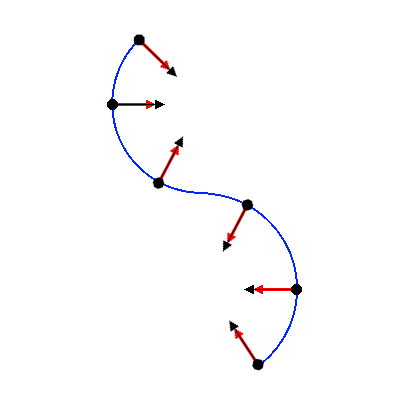Remember, when airstrafing we must always keep our acceleration perpendicular to our velocity. When holding W, our acceleration is parallel to our orientation, so if we are going to W strafe we much look into our turn, specifically, we must look at the center of our turn. This is demonstrated in the figure to the right.

There are two problems that should jump out at you here: First, we're not looking where we're going, the largest field of view we can get is 53 degrees on either side [Note 2], but we are looking 90 degrees away from forwards. This makes it very hard to steer or react to changes on the battlefield.

Second, there is a sudden shift of orientation when we change directions. We must turn 180 degrees in an instant. This is much harder than simply switching from A to D. An alternative would be to switch from W to S, so that we face away from the center of our turn, but this makes it even harder to see where we're going.

Note that the techniques described below are fairly advanced. You should not intentionally W strafe until you are entirely comfortable with the standard A and D strafing, as this will only confuse your muscle memory and could cause you to develop bad habits.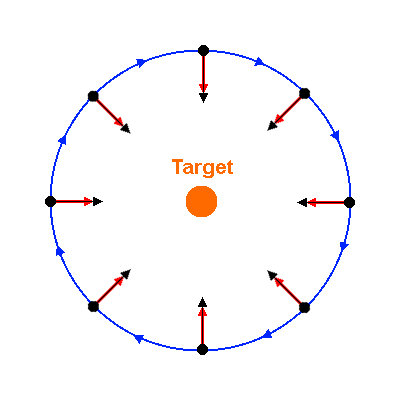So why would we ever want to W strafe? Well, sometimes we might want to look at the center of our turn. We may be bombing a medic from high above, or maybe we've been popped into the air by a soldier, in either case we want keep the target in out sights while dodging return fire. W strafing allows us to circle our target, making us very difficult to hit, while keeping him in the center of our screen. A and D strafing would either force us to look away from the target, or not let us properly circle.

Other times, we may not have a choice in how we strafe. When a demoknight charges, he is locked into "holding" W for the duration of the charge, this holds even if he launches into the air by trimping off a ramp. Therefore it is necessary for the professional demoknight to master W strafing. Let's say that you have charged into the air, and want to pick the enemy medic. The natural thing to do is to look towards the medic, but what happens if we do this? Well just as above, we circle the medic!

But unlike above, we don't want to circle the medic, we want to hit and connect with our melee. So how can we do that? The trick is to look further into the turn, so that the center of our circle moves and our path connects with the medic: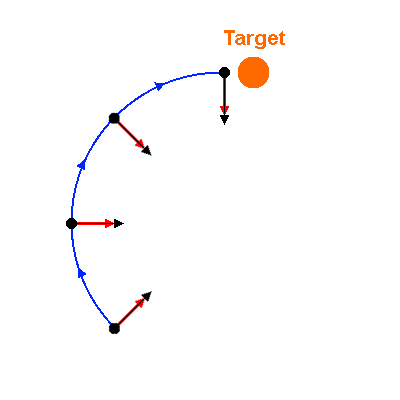When trimping we must also be careful to change our direction when we touch the ground. Movement on the ground is very different than in the air (the speed cap and acceleration are much higher, and there is an additional friction factor), which means that on the ground we always want to look directly at our target. The reverse applies when leaving the ground. A demoknight needs to master these transitions to get the perfect movement.

One last technique we will consider in this section is WA and WD strafing. This is when you strafe by holding both W and A or D, while looking 45 degrees into your turn. This can be viewed as a compromise between A and D strafing and W strafing. It affords us a better view of where we're going than W strafing, while also letting us see more into the center of our turn. Changing directions requires a 90 degree turn plus swapping strafe keys. This is a fairly niche technique, but you may find it useful on occasion.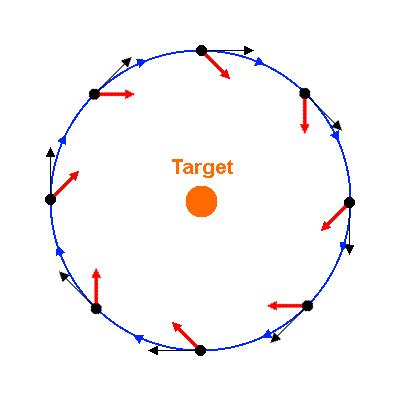Stopping
So now we know how we turn in the air. But how do we stop? If you're familiar with air strafing, you're probably well aware that pressing S in the air will bring you to a sudden stop. Let's examine this more closely.

When are looking forwards and hold S, we produce an acceleration vector opposite to our velocity. What is the projection of our velocity onto this acceleration?

|v|cos(180) = -v < L

Note that no matter how fast we're going, -v is always less than the speed limit. So the acceleration is applied and we slow down a bit. When we were turning it didn't take long for us to accelerate until the projection reached the speed limit, but here if we apply the acceleration and calculate the projection again we find that the projection is still negative, and in fact it will remain negative as long as we're still moving forwards. This means that the acceleration will quickly bring us to a stop. In fact, since acceleration is ten times the max ground speed, going from a running speed to a full stop in the air takes only 1/10 of a second.

But what happens once our velocity is zero? Well, the projetion of a 0 vector onto anything is always 0, so acceleration is applied again. But now we are moving backwards, so our velocity and acceleration are in the same direction. And since |t*a| > L for every class, we immediately reach the speed limit, which as we know, is practically standing still. This is why pressing S appears to make us stop so suddenly: It quickly removes our forward velocity, but the speed limit prevents us from accelerating in the opposite direction.

But what if we're not looking forwards? How do we stop if we've been blasted backwards, or to the side? Well remember that there is nothing special about the S key, it is the acceleration that is important. For example, if we are moving backwards, we should press W to stop. In general, we should hold the direction opposite to our movement in order to stop.
Gaining Speed in the Air
In past Team Fortress games (and many other games from that era), there was a technique called bunny hopping that allowed players to gain great speed by constantly jumping and strafing in the air, and this played an important role in these games. Sadly bunny hopping was removed in TF2 [Note 3]. But the same mechanics still exist in a much reduced form: A player can gain speed in the air by strafing.

When acceleration is added to velocity, the magnitude of the result, v', is not checked, so it is possible for v' to be larger than v. In particular, even if acceleration is perfectly perpendicular to velocity, v' is larger than v, by the Pythagorean theorem. This is analysed in more detail in the advanced section below.

The speed gained is not huge, and much of it is negated by the longer curved path you have to take to gain the speed. Since it is lost as soon as you touch the ground, it is generally not worth worrying about. For example, if you want to extend the distance of your rocket jumps, you would be better off learning how to ctap. How you air strafe should instead be determined by factors like dodging airshots or chasing down a kill.

One situation where this can be useful is if you find yourself popped into the air with very little momentum. Since your initial velocity is so low, you can gain speed relatively quickly, and this could help you avoid a follow up shot. In a situation like this, your strafes should be very tight, since this will maximize acceleration at low speeds.
For a deeper analysis of air strafing physics, we are mainly interested in two functions: The change in magnitude of velocity (change in speed) at each frame, and the change in direction at each frame. For the first, recall the equation above for v':

v' = if |v proj a| < L - |t*a|: v + t*a if L - |t*a| <= |v proj a| < L: v + (L - |v|cos(θ))*a/|a| if L <= |v proj a|: v

By applying the law of cosines to this, we can find the magnitude of v':

|v'| = if v proj a < L - |t*a|: sqrt(|v|^2 + |t*a|^2 + 2|v||t*a|cos(θ)) if L - |t*a| <= v proj t*a < L: sqrt(|v|^2 sin^2(θ) + L^2) if L <= v proj t*a: |v|

Then the change in speed is simply |v'| - |v|. Let's first take a look at how change in speed varies with angle. In this and later graphs, we will look at two typical examples, a scout at 400 HU/s (max ground speed), and a soldier at 700 HU/s (a fast speed after a rocket jump).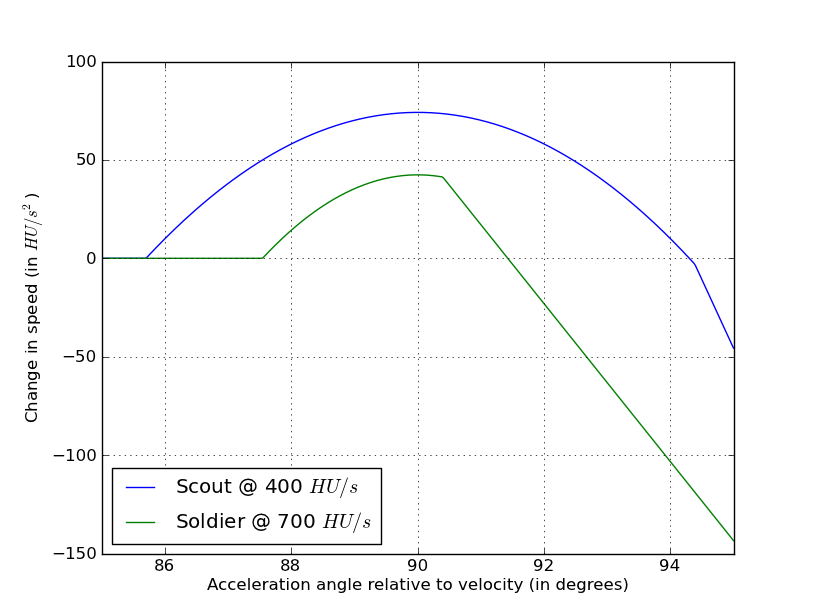Not surprisingly, the scout, with lower speed and higher acceleration, gains speed faster. We can also clearly see the three sections here: No acceleration at low angles, a smooth hump in the middle when acceleration is limited by the the speed limit, and an approximately straight line at large angles when the speed limit no longer applies.

The later first transition for the soldier is caused by his higher speed. This transition happens when L = v*cos(θ), so higher speeds require a larger angle to start accelerating. The second transition is moved forwards, both by the higher speed and the by lower acceleration. This transition happens when L - |t*a| = v*cos(θ), so higher speeds and lower acceleration cause smaller transition angles.

Most importantly, notice that the maximum increase in speed is achieved at an exactly 90 degree angle. This is true regardless of speed or class, and can easily be verified with the equation above. Let's now take a look at how change in speed depends on speed, assuming a perfect 90 degree angle is maintained.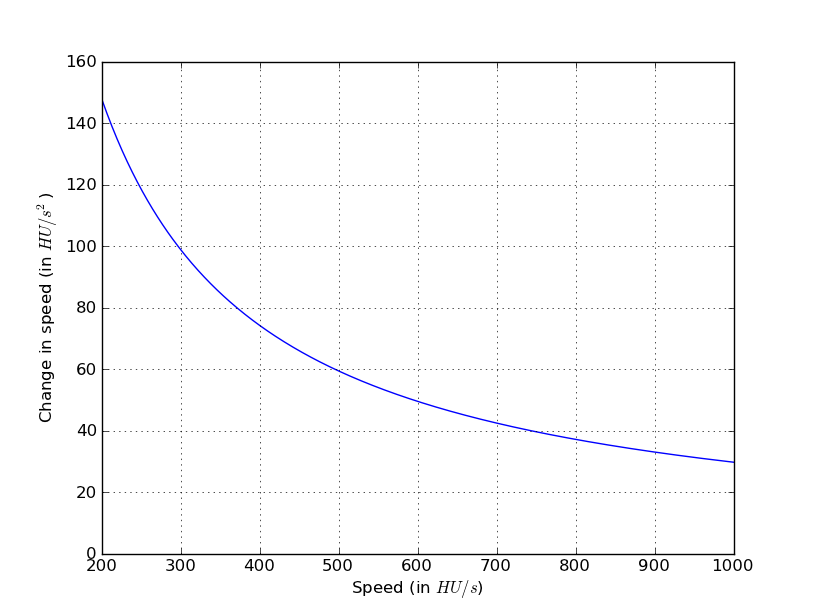We can clearly see that change in speed decreases with speed. At this angle the change in speed is simply sqrt(|v|^2| + L^2) - |v|. Since this doesn't depend on acceleration, class does not matter.

The change in direction is simply the angle between v' and v, which we will call φ. We can find this by applying the above equation to the angle definition of the dot product, v*v' = |v||v'|cos(φ).

cos(φ) = v*v'/(|v||v'|) cos(φ) = if v proj a < L - |t*a|: (|v| + |t*a|cos(θ))/sqrt(|v|^2 + |t*a|^2 + 2|v||t*a|cos(θ)) if L - |t*a| <= v proj t*a < L: (|v| + L*cos(θ) - |v|cos^2(θ))/sqrt(|v|^2 sin^2(θ) + L^2) if L <= v proj t*a: 0

Let's first take a look at φ as a function of θ: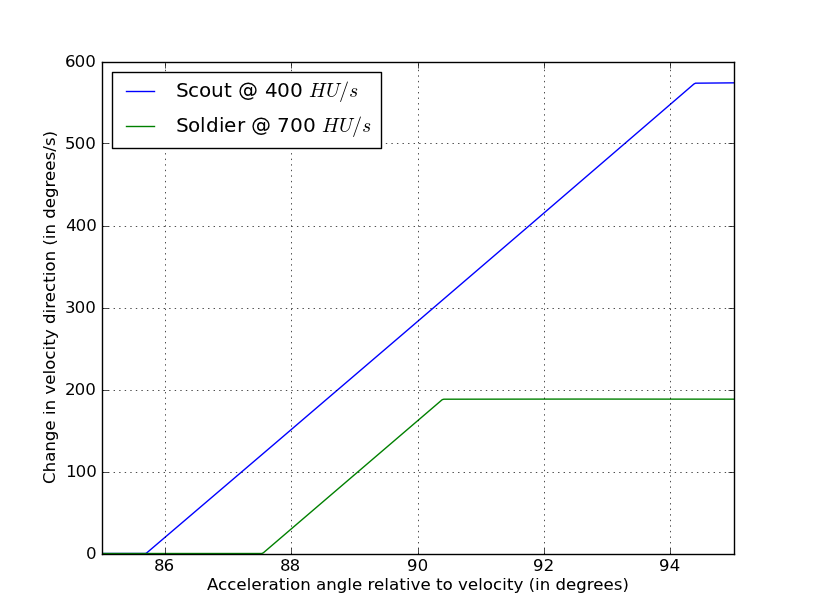The three sections are again clearly visible. At low angles, we don't turn at all. At larger angles, turning increases approximately linearly, but is limited by the speed limit. At larger angles still, the turn is approximately constant (though not quite).

We can find the maximum possible turn with calculus, or by noting that in the non-limited case (v proj t*a <= L - |t*a|), the maximum turn occurs when v' is perpendicular to a. Then θ = cos(-|t*a|/|v|) and φ= asin(|t*a|/|v|). This is shown here. We can substitute this back in to the limit equation to verify that this is allowed, and we get,

L - |t*a| >= |v|*cos(θ) (L - |t*a|)/|v| >= cos(θ) = -|t*a|/|v| L - |t*a| >= -|t*a| L >= 0

Which is of course true, so we don't need to worry about the other cases. Therefore the maximum possible turning rate is asin(|t*a|/|v|)/t degrees per second, and it is achieved at θ = acos(-|t*a|/|v|).

There is a catch with this though: To turn at this rate, we must lose some speed. You can see this in the figure above, or by plugging θ = acos(-|t*a|/|v|) into the speed change equation. So the next logical question to ask is, how fast can we turn without losing speed? To solve this we simply check the equation for change of speed, and assuming the non-limited case, we solve sqrt(|v|^2 + |t*a|^2 + 2|v||t*a|cos(θ)) = |v|, which gives us θ = acos(-|t*a|/(2*|v|)), and then φ= 2*sin(|t*a|/(2*|v|)). (We could also have found this geometrically, as shown here). Checking the limit equation:

(L - |t*a|)/|v| >= cos(θ) = -|t*a|/(2*|v|) L - |t*a| >= -|t*a|/2 2*L >= |t*a|

If we check this for all classes, we find this is true for every class except scouts (we can see this in the graph of change in speed versus angle for scouts, above). For the scout we therefore need to take the limited case from the speed change equation. We solve sqrt(|v|^2 sin^2(θ) + L^2) = |v| and get θ = acos(L/|v|) and φ= 2*sin(L/|v|). (This can also be found geometrically, in the same way as above)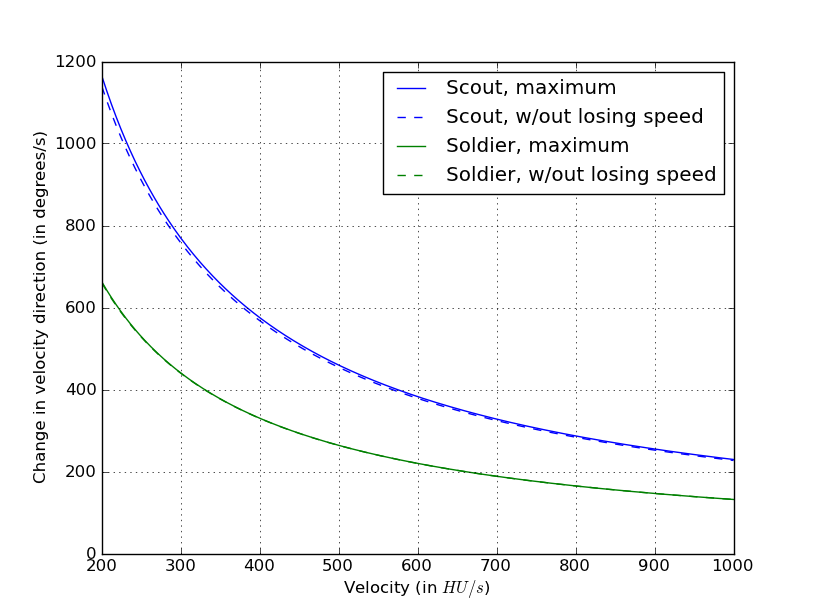But even if we know what θ we want to maintain, how can we hold this angle while turning? For most angles, this is surprisingly simple: We just turn our mouse at the turning speed. If our θ is too small, our velocity won't turn as much as we want, which will increase θ. If our θ is too large, our velocity will turn faster than we want, which will decrease θ. Therefore if we want to turn at 360 degrees per second, we just move our mouse at 360 degrees per second.

The exception is if we want to maintain the maximum possible turning. In this case, if our θ is too large, our velocity will turn less than we want. This will increase θ, and therefore increase our error. As θ increases, we will lose speed. This will continue until our speed is low enough that the velocity can turn fast enough to catch up. So unless you want to lose speed, give yourself some room for error when turning.
Have you ever seen a demoknight hit a ramp and fly across the map? A trimping demoknight can go much further than a grounded one, and there is a reason for that. When a demoknight is charging, his ground speed is 750 HU/s (637.5 with the Scotsman's Skullcutter), which means that his acceleration is 7500 HU/s^2. But most importantly, the speed limit L also increases to 750. A demoknight charge is the only thing in the game that can increase L. The same movement formulas as above still apply, but this large L value has some significant effects.

Previously, because typically L < |t*a|, the maximum change in speed was gained at a perpendicular angle. But in this case L > |t*a|, so the maximum angle is achieved when

v proj a = L - |t*a| or
|v|*cos(θ) = L - |t*a|

Therefore, the optimal angle for acceleration is θ = acos((L - |t*a|)/|v|), and change in speed at this point is (sqrt(|v|^2 - |t*a|^2 + 2|t*a|L) - |v|)/t.

Let's see what this looks like. First, let's consider acceleration with respect to angle: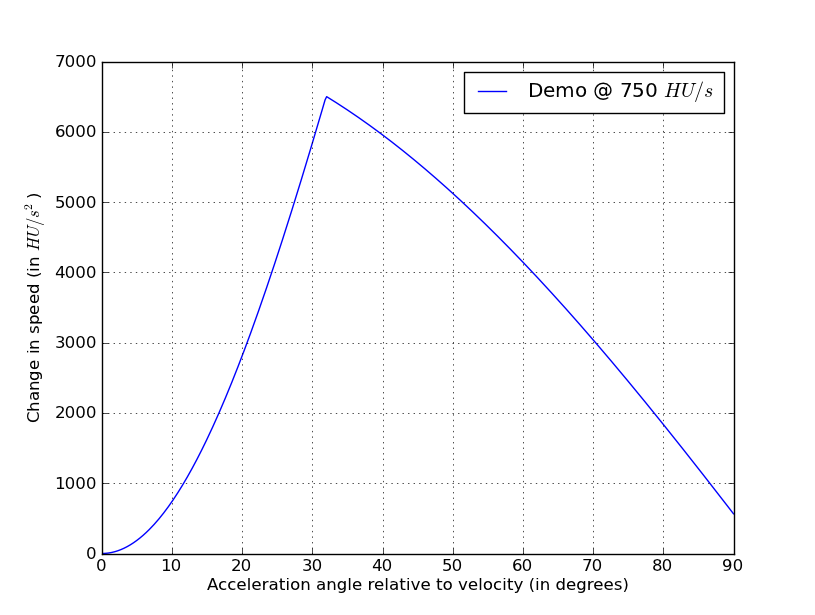Note that unlike the previous graphs, this one goes from 0 degrees (looking forwards) to 90 degrees (looking perpendicular). The maximum acceleration is nearly 6500 HU/s^2 at a 32 degree angle. This is enormous.

Now let's see how this acceleration changes with speed: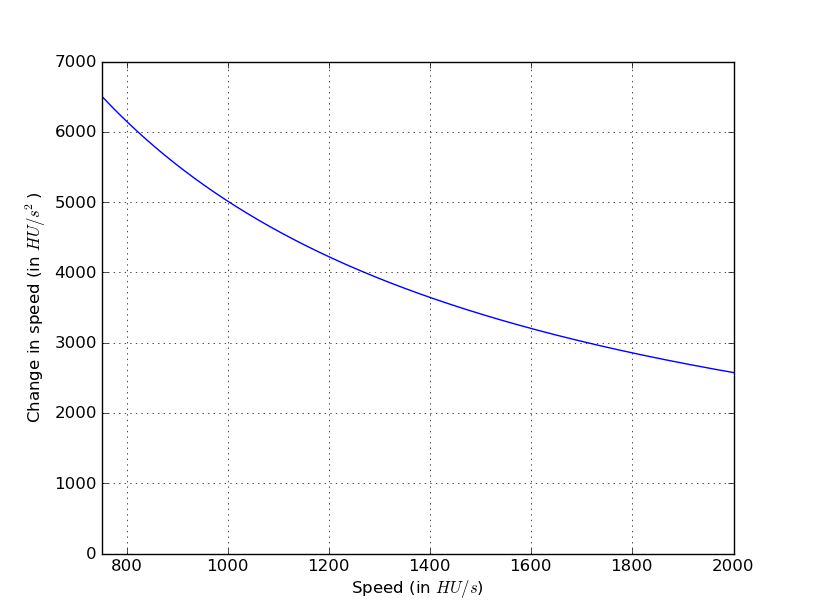The falls off pretty quickly as speed increases, but still remains enormously large compared to what we saw before. This is why a trimping demoknight can seem to travel so far once he's in the air: He is actually rapidly accelerating above typical speeds.

Finally, let's look at the relation between optimal angle and speed. We'll also include the minimum angle require to gain speed, which we discussed at the beginning of this guide: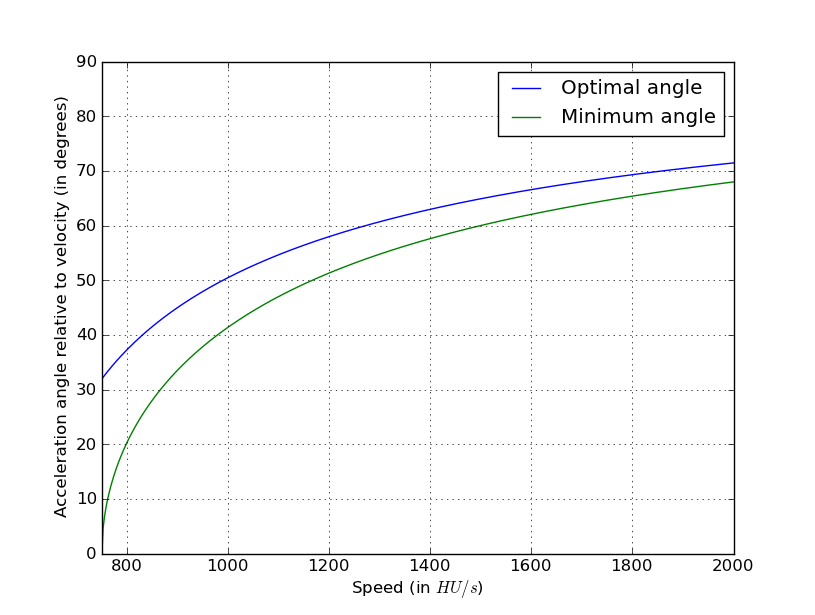We can see here that gaining speed is initially easy, occurring at any angle greater than 0 and maximized at a small angle, but both the minimum and optimal angles quickly increase with speed. At higher speeds, further gains require much sharper turning, although still less than an ordinary class.
Credits
Inspired by "Strafing Theory" by injx, a mathematical guide to strafe jumping in Quake 3. The Source engine is distantly derived from the Quake engine, and the "rules" of movement are basically the same, but many of the constants (the speed limit and air acceleration, to name a few) are very different.

Notes
Note 1: I determined the air acceleration values experimentally. These are the steps to reproduce the experiment:

1. Choose a map with large open air space. I used jump_pagoda for this experiment.
2. Choose a class and loadout to test.
3. Go to a high ledge or cliff.
4. Set sv_gravity 0.
5. Set cl_showpos 1. This will show your velocity in the top right corner.
5. Walk off. Allow yourself some time to move away from the ledge or cliff.
6. In the console, type "+forward; +left"
7. Choose a value of cl_yawspeed to test. cl_yawspeed is your turning rate in degrees per second.
8. Allow some time for your speed to settle. It should either start fluctuating in a small range around some value, or asymptotically approach some value.
9. Record the cl_yawspeed and the velocity.
10. Repeat steps 7 through 9 to gather a variety of datapoints.
11. Repeat steps 2 through 10 for different classes and loadouts, as desired.

Then given a cl_yawspeed θ and steady state velocity v, the acceleration can be calculated as:
a = 2*pi*v*θ/360.

This can be derived with vector calculus, or you can observe that in the steadystate, the acceleration turns the velocity vector in a circle without changing it's magnitude. The circumference of this circle is 2*pi*v, and the velocity vector moves with speed a (by the definition of acceleration), so it takes 2*pi*v/a seconds to complete a circle. The acceleration vector is turning at θ degrees per second, so it takes 360/θ seconds to turn a complete circle. Because this is a steady state, the velocity and acceleration vectors both turn a complete circle in the same time, so 2*pi*v/a = 360/θ, and solving for a gives 2*pi*v*θ/360 = a.

For a single class and loadout, your calculated acceleration values should be close to the same. The data I gathered was:

Heavy: θ v a 100 1317.5 2299 200 659 2300 400 330 2304 800 165 2304 Heavy w/ GRU: θ v a 100 1713 2990 Scout θ v a 100 2291 4000

If you want to experiment or just have fun with air strafing, the above technique of walking off a ledge with sv_gravity 0 is a good way to do it. See how fast you can go while maneuvering around obstacles on your favorite map!

Note 2: The field of view on a widescreen monitor is larger than the fov setting would indicate. For a 90 field of view setting on a 16:9 monitor, the actual horizontal FOV is about 106. (http://forums.steampowered.com/forums/showpost.php?p=34851556&postcount=2)

Note 3: Bunny hopping existed very early in TF2, but was fixed in the October 31, 2007 patch. Since then, when a player moving faster than the ground speed lands, he loses any additional speed, so jumps cannot be chained together to build and keep high speeds.

...Except not quite. It turns out, it is still barely possible to bunny hop, as shown in this video. This isn't something you need to try to master though.
the coolest Apr 12 @ 11:17pm
i wanna say: ive been learning gamedev and wanted to figure out how source air control actually works, and i haven't found an actual explanation until now. you are an absolute lifesaver!!!
DOOT Feb 10 @ 9:53pm
Quake physics be like:
Shoota Jan 9 @ 4:12pm
w strafing with the d or a button are good ways to fool the opposing team. It works good for getting away, around, or through enemy players. btw this is the best strafing guide I have seen. W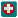Kered13  [author] Nov 21, 2021 @ 8:09pm
Double jumps: I assume you're talking about the Scout. Double jumping with the Scout immediately sets your speed to 400 hu/s (horizontal) in the new direction. It ignores all of these equations.

Terminal velocity: I believe there is a terminal velocity but I'm not sure what it is, except that it's pretty high. I could be wrong though.

Other games: The same equations apply in every Source engine game before TF2. I believe it applies to both L4D games and CS:GO. It applies in Portal 2 *except* that if your horizontal speed is too high (I'm not exactly sure but something like 150% normal speed) your air acceleration is set to 0, preventing any further turning.

Z-axis: The Z-axis can be ignored. More specifically, the player's input direction (which determines the air acceleration direction) is always in the horizontal plane, so when velocity is projected onto the air acceleration direction the vertical component disappears. Gravity is applied separately from air acceleration.
Laser Bread Nov 21, 2021 @ 2:27pm
Also, does this speed limit care about the z-axis? Like, can you not accelerate if you're falling at a certain speed.
Laser Bread Nov 21, 2021 @ 2:23pm
I think the purpose of Valve making the acceleration limit this way is so that players can stop in midair, slightly influence where they're going, but not be able to yeet off in a different direction entirely.

So, if that's the case, then how do midair jumps get immunity to the acceleration limit? You can jump in one direction, than double jump in the opposite direction. How does that work?

One final question I have: is there a terminal velocity in the TF2, and has airstrafing been fixed in future FPS Source games (L4D2, Portal 2, etc)?
Kered13  [author] Oct 31, 2021 @ 11:45am
@879m: The graph is correct, remember that the equation is for the change per tick while the graph is for the change per second , so you need to multiply by 66.
DroXi Jun 29, 2021 @ 1:17pm
"How to become an astronaut"
879m May 10, 2021 @ 8:50am
For the second graph in the equations of motion section (change in speed vs speed) I get completely different values to yours using Δv = sqrt(|v|^2| + L^2) - |v|. Is that not the correct formula? To match your numbers I have to increase L from 30 to ~252.
Freakzone12 Apr 29, 2021 @ 9:31am
Thank you for doing this bro